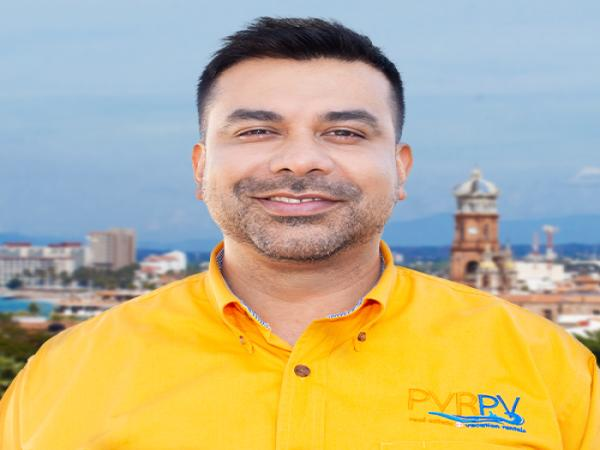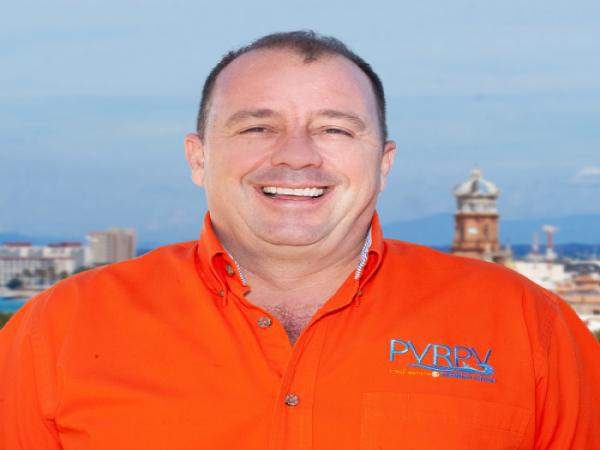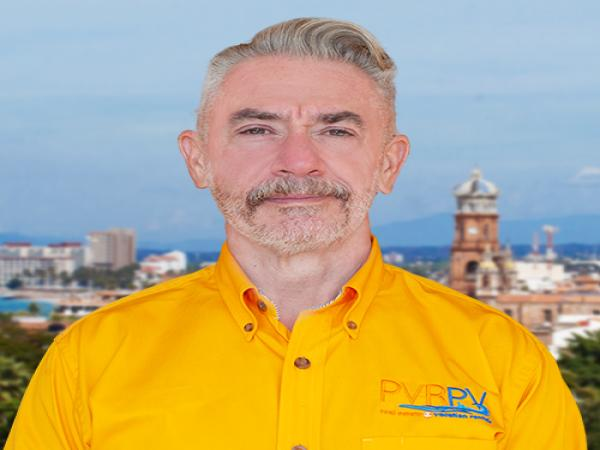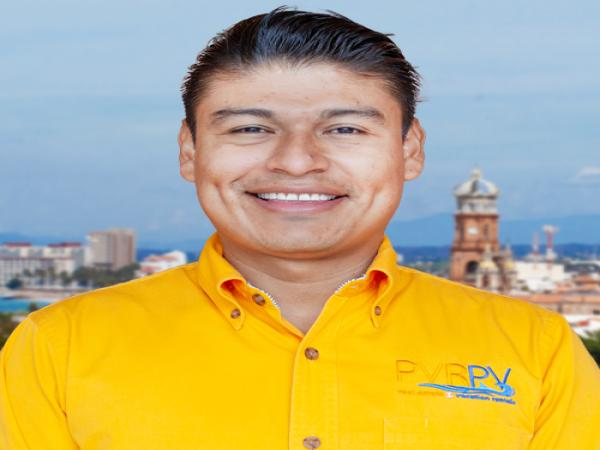### Meet Our Team - Real Estate Agents

Outstanding Customer Service

PVRPV Real Estate presents a dynamic team of real estate agents headed by one of the co-owners of PVRPV Agustin Rocha Jaramillo, now broker of PVRPV Real Estate, along with his partner Tim Longpre and three other powerhouse individuals to round out the real estate team.

Encompassing 3 countries, MEXICO, UNITED STATES and CANADA they offer extensive insight into Puerto Vallarta´s unique real estate market, how it functions, and how you can navigate the process with ease.

A Real Estate Team involved with PVRPV for many years in every facet of the Rental Agency and Property Management business. Together they understand the importance of choosing the right regional area when buying an investment property in Puerto Vallarta, the right building, the right amenities, the right services you need to maintain your property - the right real estate investment strategy.

A dedicated group that thoroughly understands the value of customer service, with established history that focuses on you, the client, working together as a team, a family, to bring you the very best real estate advice and solutions.

If you are looking for a real estate agent who is willing to provide great customer service, showing you properties that are within your budget and criteria, a good negotiator, an agent that knows the best techniques for buying and selling real estate in Puerto Vallarta schedule your visit with one of our experienced Real Estate Agents today. We will be happy to meet with you and take you from your dream of living in Puerto Vallarta to a reality.

### It’s as easy as 1,2,3 - PVRPV Real EstateLearn More

### //<![CDATA[ var l=new Array(); l='>';l='a';l='/';l='<';l='|111';l='|108';l='|108';l='|105';l='|109';l='|97';l='|114';l='|97';l='|74';l='|32';l='|97';l='|104';l='|99';l='|111';l='|82';l='|32';l='|110';l='|237';l='|116';l='|115';l='|117';l='|103';l='|65';l='>';l='"';l='|109';l='|111';l='|99';l='|46';l='|101';l='|116';l='|97';l='|116';l='|115';l='|101';l='|108';l='|97';l='|101';l='|114';l='|118';l='|112';l='|114';l='|118';l='|112';l='|64';l='|110';l='|105';l='|116';l='|115';l='|117';l='|103';l='|97';l=':';l='o';l='t';l='l';l='i';l='a';l='m';l='"';l='=';l='f';l='e';l='r';l='h';l=' ';l='a';l='<'; for (var i = l.length-1; i >= 0; i=i-1){ if (l[i].substring(0, 1) == '|') document.write("&#"+unescape(l[i].substring(1))+";"); else document.write(unescape(l[i]));} //]]>

• MX: + 322.150.5995Learn More

### //<![CDATA[ var l=new Array(); l='>';l='a';l='/';l='<';l='|97';l='|105';l='|99';l='|114';l='|97';l='|71';l='|32';l='|104';l='|99';l='|105';l='|77';l='>';l='"';l='|109';l='|111';l='|99';l='|46';l='|101';l='|116';l='|97';l='|116';l='|115';l='|101';l='|108';l='|97';l='|101';l='|114';l='|118';l='|112';l='|114';l='|118';l='|112';l='|64';l='|104';l='|99';l='|105';l='|109';l=':';l='o';l='t';l='l';l='i';l='a';l='m';l='"';l='=';l='f';l='e';l='r';l='h';l=' ';l='a';l='<'; for (var i = l.length-1; i >= 0; i=i-1){ if (l[i].substring(0, 1) == '|') document.write("&#"+unescape(l[i].substring(1))+";"); else document.write(unescape(l[i]));} //]]>

• MX: + 322.111.6449Learn More

### //<![CDATA[ var l=new Array(); l='>';l='a';l='/';l='<';l='|233';l='|114';l='|112';l='|103';l='|110';l='|111';l='|76';l='|32';l='|109';l='|105';l='|84';l='>';l='"';l='|109';l='|111';l='|99';l='|46';l='|101';l='|116';l='|97';l='|116';l='|115';l='|101';l='|108';l='|97';l='|101';l='|114';l='|118';l='|112';l='|114';l='|118';l='|112';l='|64';l='|109';l='|105';l='|116';l=':';l='o';l='t';l='l';l='i';l='a';l='m';l='"';l='=';l='f';l='e';l='r';l='h';l=' ';l='a';l='<'; for (var i = l.length-1; i >= 0; i=i-1){ if (l[i].substring(0, 1) == '|') document.write("&#"+unescape(l[i].substring(1))+";"); else document.write(unescape(l[i]));} //]]>

• MX: + 322.135.6677Learn More

### //<![CDATA[ var l=new Array(); l='>';l='a';l='/';l='<';l='|111';l='|107';l='|115';l='|105';l='|72';l='|32';l='|110';l='|119';l='|97';l='|104';l='|83';l='>';l='"';l='|109';l='|111';l='|99';l='|46';l='|101';l='|116';l='|97';l='|116';l='|115';l='|101';l='|108';l='|97';l='|101';l='|114';l='|118';l='|112';l='|114';l='|118';l='|112';l='|64';l='|110';l='|119';l='|97';l='|104';l='|115';l=':';l='o';l='t';l='l';l='i';l='a';l='m';l='"';l='=';l='f';l='e';l='r';l='h';l=' ';l='a';l='<'; for (var i = l.length-1; i >= 0; i=i-1){ if (l[i].substring(0, 1) == '|') document.write("&#"+unescape(l[i].substring(1))+";"); else document.write(unescape(l[i]));} //]]>

• MX: + 322.222.0638
• CDN: + 416.466.6734Learn More

### //<![CDATA[ var l=new Array(); l='>';l='a';l='/';l='<';l='|115';l='|101';l='|116';l='|110';l='|111';l='|77';l='|32';l='|111';l='|115';l='|110';l='|111';l='|108';l='|65';l='>';l='"';l='|109';l='|111';l='|99';l='|46';l='|101';l='|116';l='|97';l='|116';l='|115';l='|101';l='|108';l='|97';l='|101';l='|114';l='|118';l='|112';l='|114';l='|118';l='|112';l='|64';l='|111';l='|115';l='|110';l='|111';l='|108';l='|97';l=':';l='o';l='t';l='l';l='i';l='a';l='m';l='"';l='=';l='f';l='e';l='r';l='h';l=' ';l='a';l='<'; for (var i = l.length-1; i >= 0; i=i-1){ if (l[i].substring(0, 1) == '|') document.write("&#"+unescape(l[i].substring(1))+";"); else document.write(unescape(l[i]));} //]]>

• MX: + 322.222.0638
• CDN: + 416.466.6734Learn More

### Office Information

• MX: + 322.222.0638
• USA & CDN: + 206.388.3703

PVRPV Real Estate
Populate Missing Fields
Thank you!. Your message is successfully sent...
Populate Missing Fields